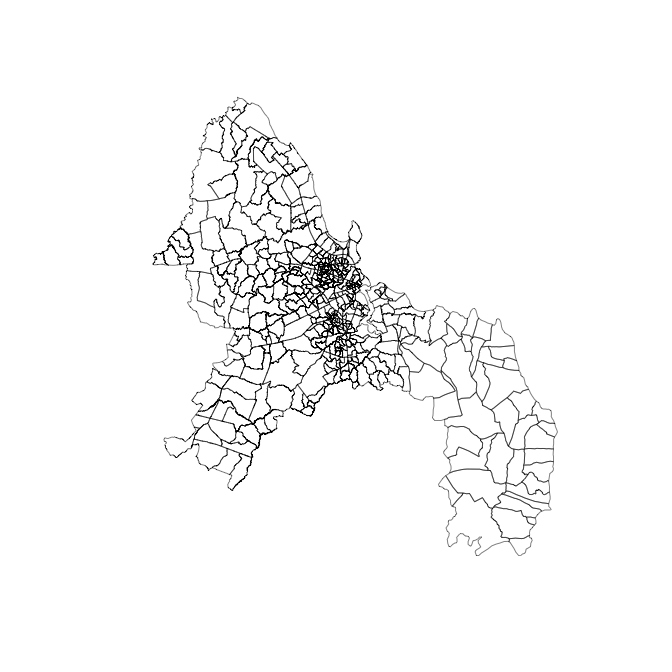# BSBT: A simple example

BSBT (Bayesian Spatial Bradley–Terry) is a package which fits a Bradley–Terry model with a spatial component for comparative judgement data sets. This can be used to estimate the quality of objects used in the data set.

In this vignette, we’ll go though a simple example, using the package to simulate comparative judgement data and infer model parameters from the data. This vignette will use the following packages:

# Simulating data

In this vignette, we’ll use the example of deprivation in Dar es Salaam, Tanzania. The city has 452 subwards and we will generate a deprivation level for each subward. We will then simulate a comparative judgement data set, where the subwards are compared based on their deprivation level. The package includes shapefiles for the 452 subwards in Dar es Salaam. We load the shapefiles and adjacency matrix for the city by calling

data("dar.adj.matrix")
data("dar.shapefiles")
plot(dar.shapefiles$geometry, lwd = 0.5)The $$(i,j)^{th}$$ element of the adjacency matrix is 1 if subwards $$i$$ and $$j$$ are neighbors and 0 otherwise. We manually added two extra pairs of neighbors to allow for connections across the Kurasini creek, which flows through the city. ## Simulation The aim of a comparative judgement study is to estimate the quality of each object in the study; in the example, the objects are subwards and their qualities are deprivation levels. The deprivation levels can be simulated in any sensible way (e.g. from a uniform or normal distribution). In this example, we will draw the deprivation levels from a multivariate normal distribution, where the deprivation levels are spatially correlated. To generate the covariance matrix for the multivariate normal distribution, we use the matrix exponential of the city’s adjacency matrix. This assigns high correlation to highly connected subwards, and low correlation to subwards that are not well connected. We use the inbuilt covariance matrix function to generate this k <- constrained_adjacency_covariance_function(dar.adj.matrix, type = "matrix", hyperparameters = c(1, 1), linear.combination = rep(1, 452), linear.constraint = 0) The linear combination and constraint part of this ensure that sum of the deprivation levels is 0. true.deprivation <- BSBT::mvnorm_sd(k$mean, k$decomp) ## Simulation comparisons Now we have the simulated deprivation levels, we can generate the comparative judgement data set. The code below generates 20,000 pairwise comparisons. Each comparison is a draw from a Bernoulli distribution, where the probability of success depends on the difference in deprivation levels of the pair being compared. comparisons <- BSBT::simulate_comparisons(n.contests = 20000, true.quality = true.deprivation, sigma.obs = 0) This function also outputs a win/loss matrix, where the $${i, j}$$ element is the number of times subward $$i$$ beat subward $$j$$. Setting sigma.obs = 0 means that all judges have a perfect knowledge of the deprivation levels in the city. Changing this value adds noise the deprivation levels used in each comparison. # Inference We can use the simulate data to infer the deprivation levels. The following code runs the MCMC algorithm and produces a set of samples from the posterior distribution: set.seed(123) mcmc.output <- run_mcmc(n.iter = 300000, delta = 0.01, covariance.matrix = k, comparisons$win.matrix, f.initial = rep(0, 452), alpha = FALSE)

This may take 40 minutes to run, so it is not run in this vignette. It runs the MCMC algorithm for one millions iterations using an initial setting all quality parameters to 0. It uses the covariance matrix k we generated when simulating the data, and sets the tuning parameter to 0.01. For this example, we do not infer any hyperparameters related to k and so set alpha = FALSE.

To obtain the results, we set a burn in period of 30,000 iteration (10% of the total number of iterations) and compute the posterior mean and 95% credible intervals:

estimated.deprivation <- colMeans(mcmc.output$f[-c(1:30000), ]) upper.deprivaiton <- apply(mcmc.output$f[-c(1:30000), ], 2, quantile, 0.975)
lower.deprivaiton <- apply(mcmc.output\$f[-c(1:30000), ], 2, quantile, 0.025)

We can check the true deprivation levels are correctly inferred with the following plot

plot(true.deprivation, estimated.deprivation)
segments(x0 = true.deprivation, y0 = lower.deprivaiton, y1 = upper.deprivaiton)
abline(0, 1)

All the credible intervals span the line $$y = x$$.